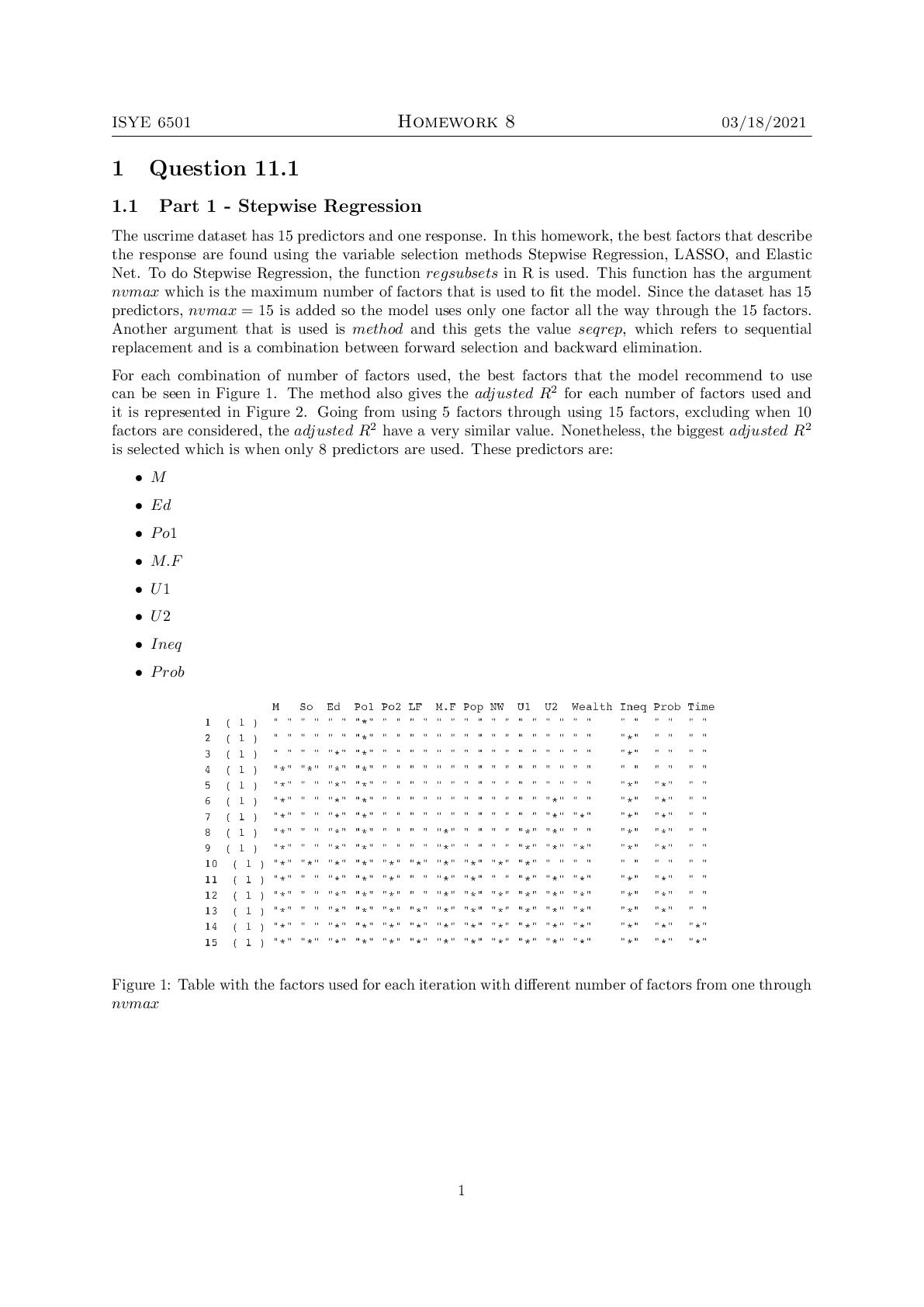Education > Questions and Answers > ISYE 6501X : Homework_8 Regression

# ISYE 6501X : Homework_8 Regression

Part 1 - Stepwise Regression The uscrime dataset has 15 predictors and one response. In this homework, the best factors that describe the response are found using the variable selection methods Stepwise Regression, LASSO, and Elastic Net. To do Stepwise Regression, the function regsubsets in R is ... used. This function has the argument nvmax which is the maximum number of factors that is used to t the model. Since the dataset has 15 predictors, nvmax = 15 is added so the model uses only one factor all the way through the 15 factors. Another argument that is used is method and this gets the value seqrep, which refers to sequential replacement and is a combination between forward selection and backward elimination. For each combination of number of factors used, the best factors that the model recommend to use can be seen in Figure 1. The method also gives the adjusted R2 for each number of factors used and it is represented in Figure 2. Going from using 5 factors through using 15 factors, excluding when 10 factors are considered, the adjusted R2 have a very similar value. Nonetheless, the biggest adjusted R2 is selected which is when only 8 predictors are used. These predictors are: Part 2 The next method used is LASSO. In R, the function cv:glmnet and glmnet are used to cross-validate and train a LASSO model respectively. This function is also used to train a model using Elastic Net. The model receives a parameter called alpha, when given the value of one, uses the LASSO method. It also has another parameter called lambda and using cross-validation the best lambda is selected. The function has as default 100 as the numbers of lambda to be tested, but this parameter is changed for 50 since the rst (263.095) and last lambda (0.0383) tested are the same between the two and only the amount of lambdas between those two values change so the nal result is practically the same. To compare how the folds did with the di erent values of lambda, the function returns the mean cross- validated errors (CVM) and this is represented in Figure 4. Lambdas values goes from 263.095 to 0.0383, so as lambda gets smaller, the error also gets decreases. Due to the nature of cross-validation, the possibility of the best lambda given by the lowest error being selected randomly is very high. For this, the biggest lambda with an error that is one standard error away from the smallest is chosen. This lambda is 27.575 with an CVM of 77170.12. [Show More]Price:

#### \$10.00

Pages:

27 pages

Rating:

517
0master papers
Joined: 3 years ago
Papers sold: 253

##### Written for

Category:

Course:

Education

Last updated at :

8 months ago

Language
English